SEARCH HOMEMath Central Quandaries & QueriesQuestion from Pamela: We need to mark 1/2 acre parcels on the property at the following attachements. [link to GIS system] Total acreage is 11.93 acres.  We need to divide the property into 1/2 acre parcels beginning from the northern border. (We understand that the last parcel at the southern end will be less than 1/2 acre ... that is okay) We are using a parcel map that has a legend of 100' = 1" If you could tell us how far to measure down on each the Western boarder and the Easter boarder to draw the southern boarder of each 1/2 acre parcel that would be wonderful. Thank you very much for your assistance.Hi Pamela.

I used the GIS software on the web site you indicated to measure the distances in feet of the irregular pentagon that your property is bounded by, as well as two diagonals, necessary for determining the actual angles involved. Hence, the calculations should be pretty close, but there is some approximation due to not knowing the actual (or relative) GPS locations of the corners.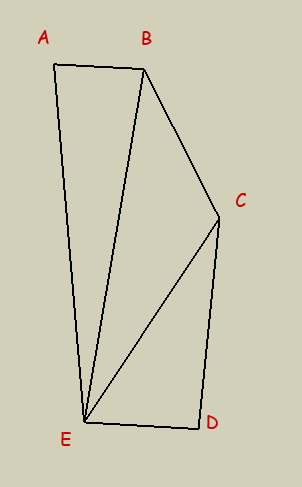Measurements:
AB = 315', BC = 582', CD = 735', DE = 397', EA = 1252'
BE = 1248', CE = 849'
AB is parallel to DE.

I measured the diagonals so that I can use the Law of Cosines to find the measures of any angles I want.

For angle BAE, this is:
BE2 = AB2 + EA2 - 2(EA)(AB)cos(BAE)

which makes angle BAE = 82.04 degrees.

Using the same technique,

angle CBA = 119.47
angle DCB = 148.27
angle EDC = 92.26
angle AED = 97.96

My understanding is that you want 1/2 acre parcels with opposite sides parallel to AB and DE. Since we are working with feet, let's convert 1/2 acre to 21780 square feet.

So we are going to start making a series of trapezoids with this area starting from the top. To deal with the corner at C, the easiest thing to do is just draw a line through C parallel to AB (we will know the area remaining in the top trapezoid and can calculate what we want to start the lower trapezoid with).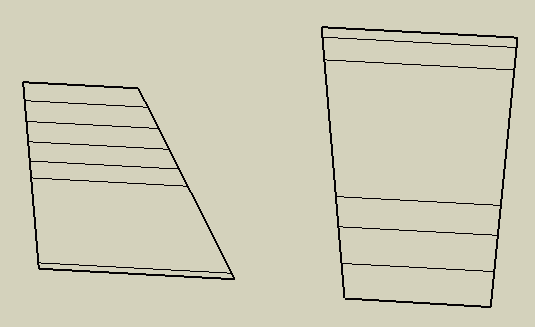Let N = the length of the north side of the first trapezoid (this is just AB).

Then let S,W,E = the length of the south, west and east segments of the same trapezoid.

Let h = the height of the trapezoid.

The area of a trapezoid is just A = ½(N+S)h. We know N and A, but need to relate S to h to solve this. To do this, I have to define a couple of other variables x and y. See the diagram below.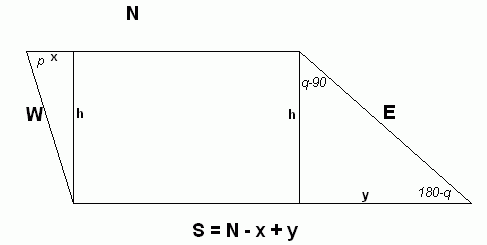Terrific! More variables we don't know! But don't despair, we'll start blending things together now. p and q are the angles BAE and CBA.

We can define x and y in terms of p, q and h using trig functions:

x = h / tan(p)
y = h / tan(180-q) = -h / tan(q)

So

S = N - x + y = N - h/tan(p) - h/tan(q) = N - h[ cot(p) + cot(q) ].

So the area of the trapezoid is calculated as:

A = ½ (N + S) h = ½(N+N - h[ cot(p) + cot(q) ] ) h.

Solving for h, we get a quadratic:

[ cot(p) + cot(q) ] h2 + (-2N) h + (2A) = 0.

Which we use the quadratic formula on: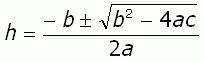where a = [ cot(p) + cot(q) ], b = (-2N) and c = (2A).

When we plug in the values p=82.04, q=119.47, N=315 and A=21780, we get two values for h, but one is ridiculous for this situation and refers to a mathematically correct, but physically impossible shape. So h = 66.19'.

Now that we have h, we can calculate W and E, which are the distances along the west and east edges down from the top.

W = h/sin(p) = 66.83',
E = h/sin(180-q) = h/sin(q) = 76.02'

So if we make a mark 66.83 feet along the west edge down from the northwest corner and join that in a line to a mark 76.02' down the east edge from the northeast corner, then we have a line parallel to the north side which is a trapezoid with the area of ½ acre.

For the next parcel, we replace the north border with the south border (we are working out way down) and do the same series of steps (it is a tedious process).

At some point we are going to come to the kink in the eastern border (point C). So when we get to the point where there is less than half an acre remaining in the top half of the large property, We will draw a line straight across from C and calculate the area of the top. That will let us calculate the area we are "missing" and we can create a small parcel to start the bottom half.

Here's what I get when I use a spreadsheet to calculate this: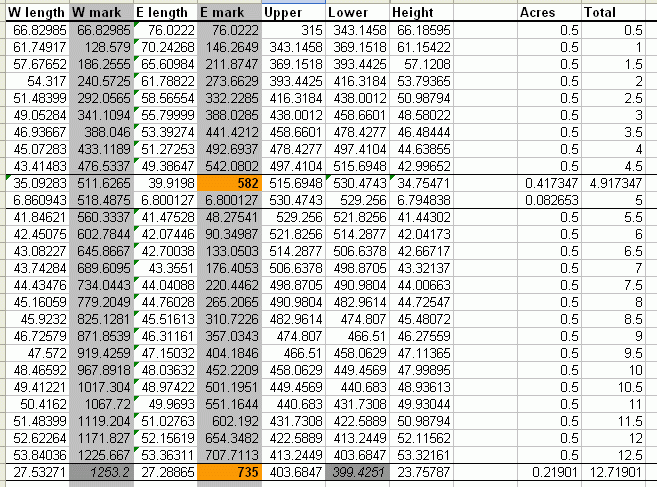The grey areas are cummulative totals along those edges. The orange items indicate where we had to use the "remainder" to make a shortened parcel. The first parcel under the first orange spot is deliberately made to be 0.082653 acres so it is combined with the one above it to make a .5 acre parcel.

The bottom parcel is the amount "left over" - about .22 acres in size. The dark grey areas indicate slight imperfections in the measurements of a couple of feet or less (these are what I referred to when I started my reply).

However, the fascinating thing about this is the cummulative total acreage! I calculate 12.72 acres, not the 11.93 you indicated and which is on the survey record! I re-calculated this area another way, and either the diagram on the GIS site is giving incorrect length measurements (which I based my calculation on) or the survey total of 11.93 is incorrect! I would guess the survey total is incorrect and you actually have more land than you thought you had.

Whew! This analysis took a long time! Hope you find it useful - send us an email if you can confirm with the county surveyor that the acreage figure is incorrect.

Regards,
Stephen La Rocque.

PS: If you want the excel spreadsheet I used, here it is.Math Central is supported by the University of Regina and The Pacific Institute for the Mathematical Sciences.